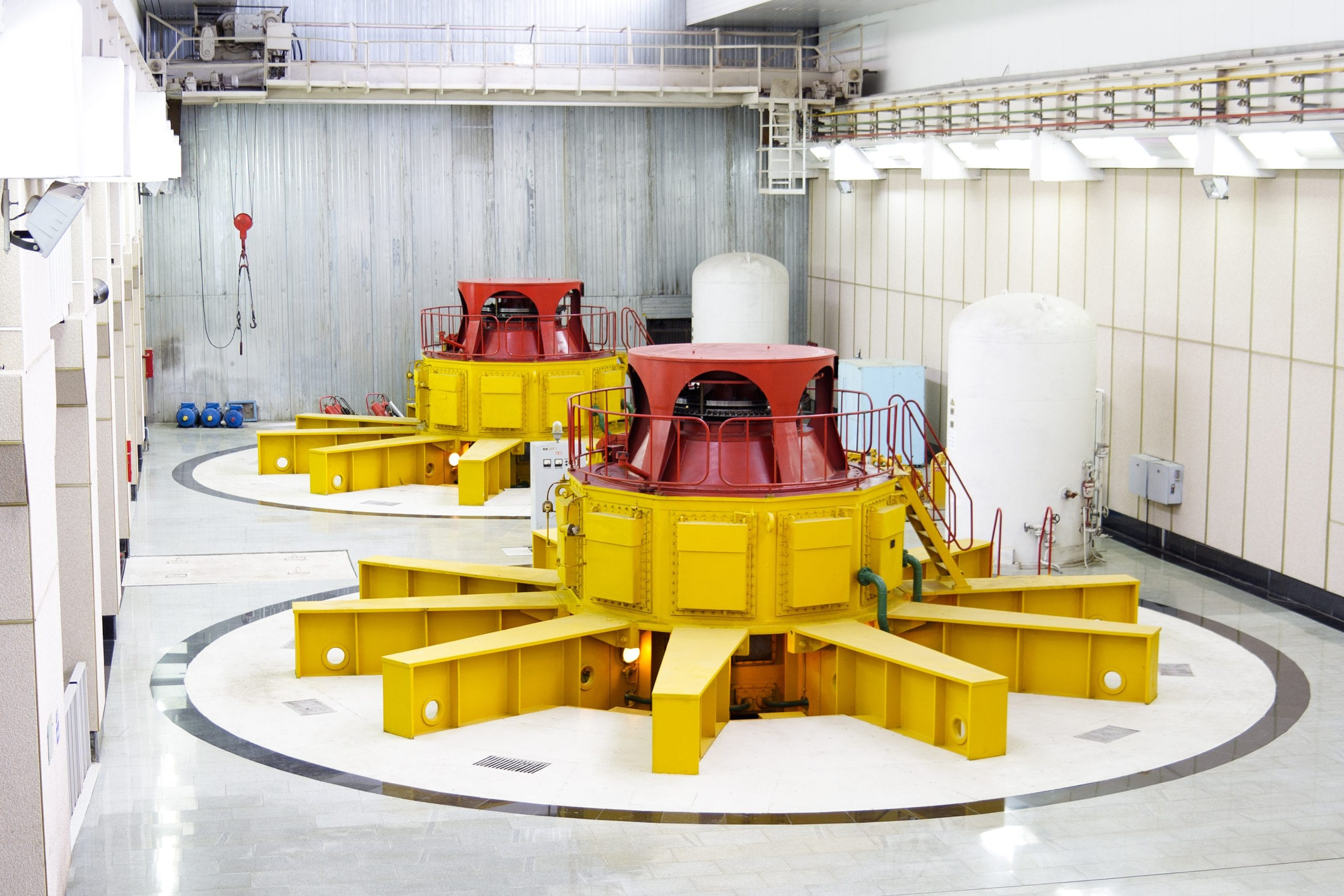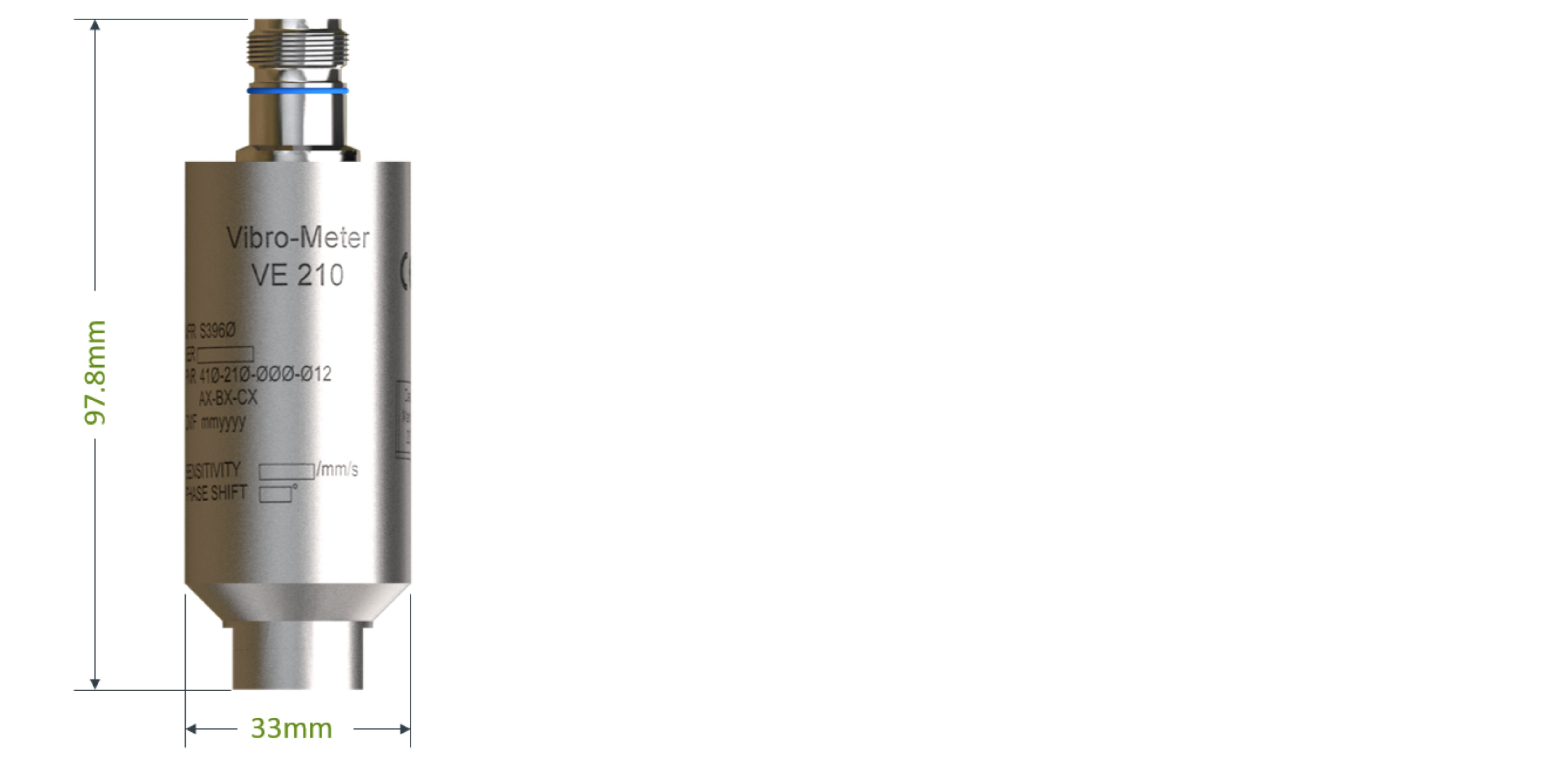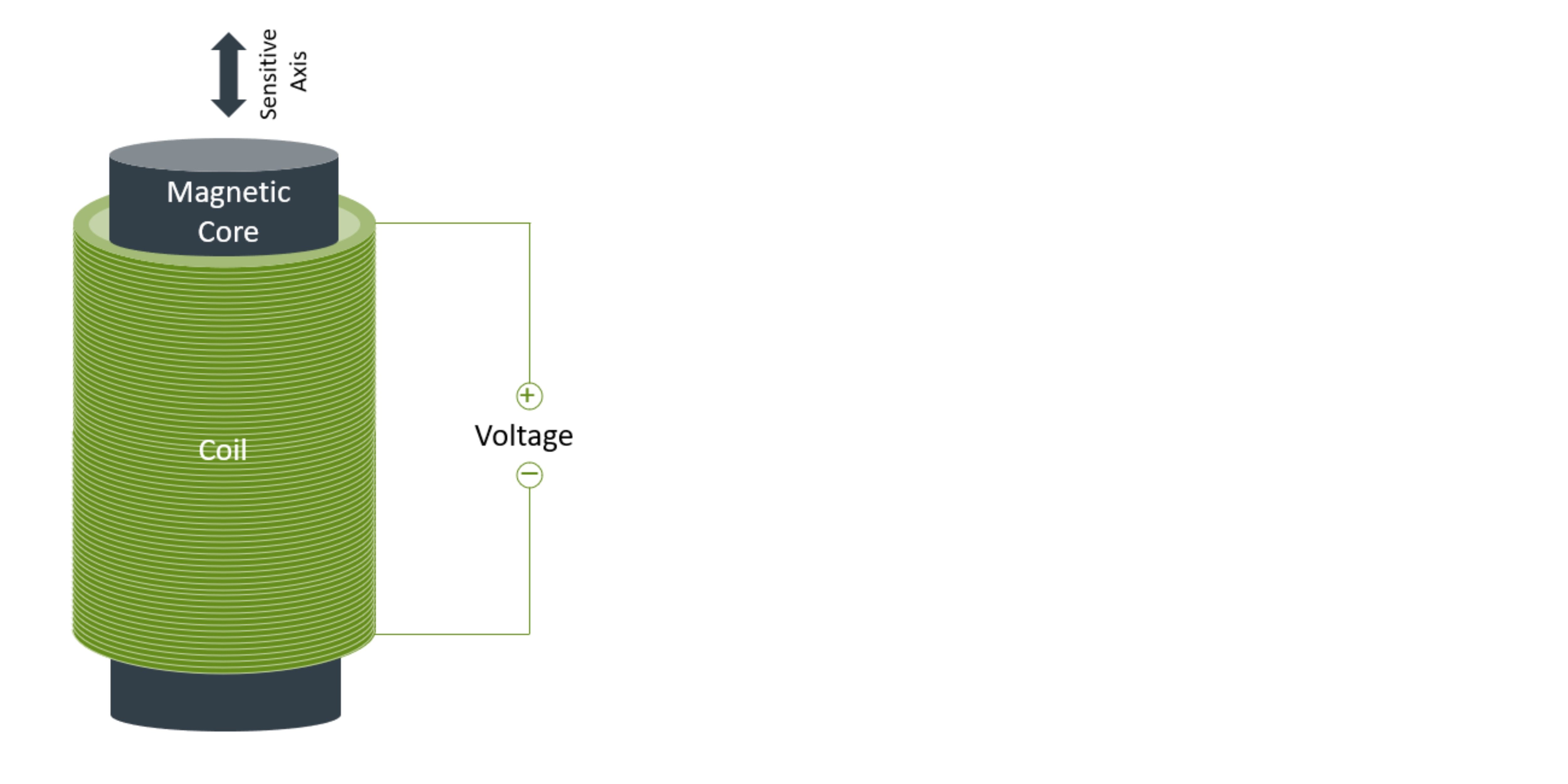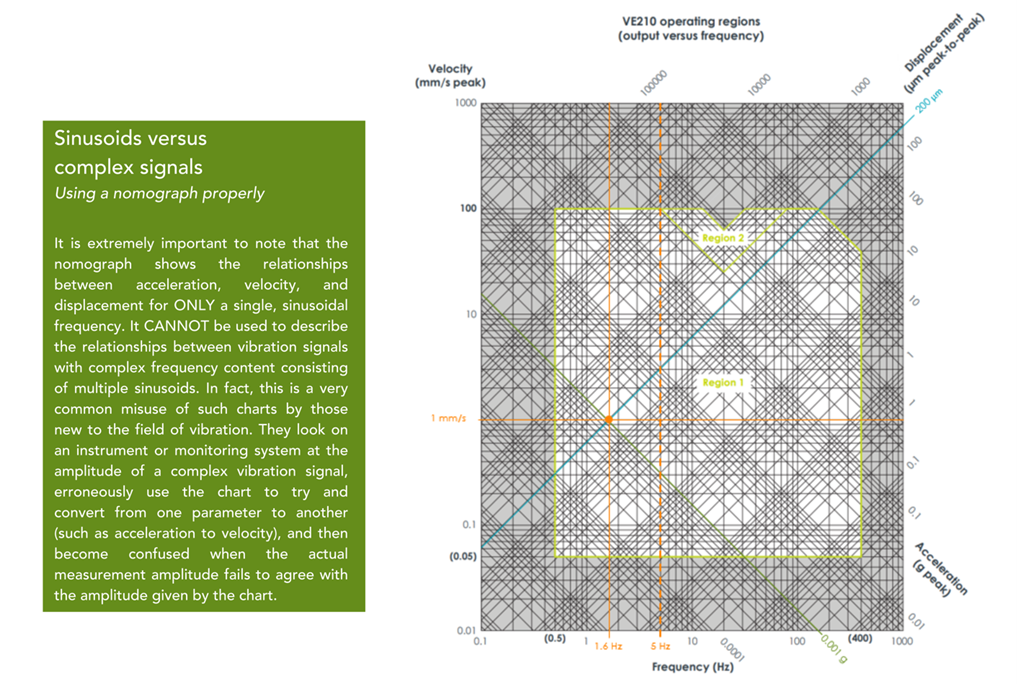# Sensor Selection Criteria for Low-Frequency Measurements – Part I## The physics of low-frequency measurements.

In this 2-part series, we examine the pros and cons of various sensors used for making low-frequency measurements. Although the hydro power sector is one of the most common industries where such measurements are required, this is by no means the only industry where such applications are found. The pulp & paper, metal, mining, cement, water treatment, and petrochemical sectors also find applications where low-frequency measurements are necessary such as on rolls, mills, rotary kilns, agitators, pelletizers, extruders, and other slow-speed machinery.

In part 1, we focus on the physics of low-frequency measurements, allowing a better understanding of why some sensors are inherently more sensitive than others and why simply mathematical integration of a signal does not automatically mean that an accelerometer is the right choice for any and all applications. In part 2, we focus on the other aspects of sensor selection and why sensitivity alone is not the sole criteria.

Introduction

Those new to the field of vibration – particularly if their learning is not reinforced with practical experience in the field – will often make the mistake of assuming that mathematical equivalencies translate into measurement equivalencies. Nowhere is this more common than in the physics of motion. More than 300 years ago, Newton showed that displacement, velocity, and acceleration are mathematically related to one another through the processes of differentiation and integration. As a refresher, the basic relationships are as follows:

v(t)=dx(t)/dt

a(t)=dv(t)/dt= d2x(t)/dt2

v(t)= ∫a(t) dt

x(t)= ∫v(t)dt= ∬a(t)dt

Where,

t = time, x(t) = displacement as a function of time, v(t) = velocity as a function of time, a(t) = acceleration as a function of time

In words, we can integrate an acceleration signal to obtain velocity; we can integrate a velocity signal to obtain displacement; and, we can double integrate an acceleration signal to obtain displacement.

Because these mathematical relationships are exact and not approximations, it is reasonable at first glance to assume that we need only a single type of sensor for all vibration measurements: an accelerometer. To obtain velocity, we merely integrate the acceleration signal and to obtain displacement we merely double integrate the acceleration signal. However, as we noted earlier, while such relationships may indeed exist mathematically, this does not mean that the practical realization of these relationships in sensor technology is flawless. Quite to the contrary, it can be fraught with practical difficulties due to the underlying physics.

This leads to a very fundamental rule-of-thumb in the vibration world: it is generally best practice to choose a sensor with a native output that matches the physical parameter you are trying to measure. In other words, if you are trying to measure displacement, select a displacement transducer, if velocity a velocity transducer, and if acceleration, an acceleration transducer.

Like many rules, there are exceptions and part of the science of vibration measurement is knowing when the exceptions are warranted and when they are not. Here, we will outline two such exceptions:

1. If the acceleration levels themselves provide a reasonably strong signal that is not close to the noise floor of the sensor, velocity can be measured via an accelerometer signal that is integrated.
2. The measurement of casing displacement (not shaft-relative displacement) is made most practically by means of a velocity sensor – not a displacement sensor.

We next examine each of these two exceptions in greater depth as they pertain to low-frequency measurements in general, and to hydro measurements in particular.

## True Velocity versus Integrated Acceleration

The only type of industrial vibration sensor providing a true (or “native”) velocity output is a moving-coil device 1Strictly speaking, laser surface velocimeters (LSVs) also measure velocity natively but are currently used only in laboratory settings – not for industrial machinery vibration measurements.. The principle of operation is quite simple and involves a coil of wire encircling a magnetic core. Relative motion between the coil and the core induces a voltage in the coil that is proportional to the relative velocity between them. It doesn’t matter whether the coil is stationery and the core moves or vice-versa. However, it is generally more practical to construct a sensor in which the coil is supported on springs and stays relatively motionless while the core itself is rigidly attached to the sensor housing and thus moves as the sensor moves2 It is indeed more appropriate to think of most “moving-coil” velocity sensors as being “moving magnet” because the design is such that the magnetic core moves with the sensor’s housing while the coil itself remains motionless. It is analogous to the suspension on a car consisting of coil springs and damping where the goal is to keep the car’s frame stationary while the wheels themselves move up and down on bumpy roads, thus keeping the passengers relatively still and comfortable.. In this manner, machinery casing vibration can be measured and the output is thus indicative of the casing or structure vibration velocity. Such sensors can be constructed to give a relatively strong output, and this is particularly true of those that measure down to low frequencies because the sensor geometry is physically large, leading to coils and magnets that are themselves relatively large and thus provide large outputs.The VE 210 is an example of a moving-coil velocity sensor.

As an example, consider vibro-meter’s VE210 velocity sensor. It is able to measure frequencies down to 0.5 Hz (30 cpm) with a sensitivity of 50 mV/mm/s (1270 mV/in/s). Considering that most commercially available velocity sensors (whether moving coil or piezo-velocity) have an output sensitivity of only 100mV/in/s – and occasionally 500 mV/in/s – the VE210 provides an output amplitude for the same excitation that is anywhere from 2.5 to 12.7 times stronger, ensuring that very small velocity levels are easily detected, even in the presence of electro-magnetic noise and interference.In a moving-coil velocity sensor, the coil and core move relative to one another and produce a voltage directly proportional to the relative velocity. They are usually constructed such that the core moves with the sensor housing while the coil is supported on springs and remains relatively motionless.

In contrast, consider so-called “velocity output” or “piezo-velocity” accelerometers that natively measure acceleration and then integrate the signal using integral electronics to produce a velocity output. The vibro-meter PV660 piezo-electric velocity sensor is such a device and provides an output of 4 mV/mm/sec at frequencies down to 1.9 Hz (114 cpm). For equivalent excitation of 1 mm/s at 1.9 Hz, the VE210 will produce an output of 50mV while the PV660 will provide an output of only 4mV. However, there is more to the picture than just the output sensitivity. To understand this, we will look at the vibration nomograph chart below.

## Reading a Vibration Nomograph

A nomograph is a graphical calculation chart introduced in the early 1900s that has primary value today for visualizing the qualitative relationships between variables rather than quantitative – given the availability of modern calculation engines such as spreadsheets and apps for computing exact values. Many times, logarithmic scales are used so that non-linear relationships become linear and thus easier to visualize. A vibration nomograph is commonly used to compute acceleration, velocity, or displacement amplitude given a particular vibratory frequency and one of the three vibration amplitudes. The chart assumes a single, sinusoidal vibration frequency – not complex vibration composed of multiple frequencies (see below).

Notice first that the orange dot corresponds to the excitation described in the preceding section. Namely, velocity of 1 mm/s (0-pk) at 1.6 Hz. Next, notice that this corresponds to a very large displacement amplitude of 200 μm (pk-pk) but an extremely small acceleration amplitude of only 0.001 g (0-pk). To put this in perspective, a typical displacement sensor (eddy current proximity chain) has a sensitivity of 4mV/μm and would give an output of 800 mV pk-pk for 200 μm of displacement. In the instrumentation world, this is a very strong signal. In contrast, consider the output of an acceleration transducer with a typical sensitivity of 100mV/g. There is only 0.001 g of vibration present which corresponds to 0.1 mV (100 μV). This is a very weak signal in the instrumentation world and will often be at or below the noise floor. Lastly, consider the outputs from the velocity sensors we conveyed earlier. For a typical piezo-velocity sensor, the output will be 3.9mV – approximately 80X lower than the output of the displacement transducer but 40X larger than the output of the accelerometer. However, when we use a moving-coil sensor such as the VE210, we get an output of 50mV which is excellent.A vibration nomograph graphically depicts the relationships between displacement, velocity, and acceleration for sinusoidal vibration at a single frequency. Here, we have shown the corresponding acceleration (green) and displacement (teal) amplitudes corresponding to a sinusoidal vibration velocity of 1 mm/s at 1.6 Hz (orange). This particular nomograph is taken from the datasheet for the vibro-meter VE210 moving-coil velocity sensor where Region 1 shows the usable range when the sensor is mounted vertically (Region 1) and when mounted horizontally (Regions 1 + 2).

What the preceding discussion shows is the limitation of the physics involved. When looking at vibration occurring at very low frequencies, displacement levels are very high while acceleration levels are very low. Velocity, on the other hand, tends to be relatively independent of frequency and indeed, this is why the velocity amplitudes are shown as horizontal lines on the nomograph – they don’t show nearly the dependence on frequency as do acceleration or displacement. Thus, no matter how low our sensor is capable of measuring, the amplitude of the acceleration itself may be so low as to be miniscule. We end up trying to measure a physical parameter that is too small at the frequency of interest and are better served by measuring a different parameter (such as velocity or displacement).
This is intuitively obvious when we think of forces. If you move your arm up and down in a flapping motion once per second, it doesn’t take much force and the displacement can be quite large – you can easily move your arm with a displacement of 50 cm once per second. However, try now to move your arm that same distance, but 1000 times per second (1 kHz). Even under the assumption that your musculature was capable of such movement (and it is not), the forces to create this displacement at this frequency would break the bones in your arm because the acceleration levels are so high. Indeed, your arm has to change direction 1000 times per second and this requires tremendous force3Recalling from physics that force (f) = mass (m) x acceleration (a), high forces for a given mass require high sinusoidal acceleration amplitudes.. It can be shown mathematically, in fact, that 50 cm of displacement at 1 kHz corresponds to more than one million g’s! Conversely, make your arm vibrate five times per second and you can probably sustain amplitudes of 1-2 cm as this corresponds to only 1g.

Machinery is subject to those same laws of physics; namely, large displacements and moderate velocity amplitudes occur at low frequencies but these correspond to extremely small acceleration levels. Indeed, as we decrease in frequency, the acceleration decreases with the square of the frequency compared to displacement. Thus, using the nomograph, a displacement of 200μm at 5 Hz corresponds to approximately 0.01 g’s while the same displacement at 1 Hz corresponds to only 0.0004 g’s (25X less). This is precisely why we tend to avoid accelerometers at very low frequencies: the acceleration levels themselves are often many orders of magnitude smaller than the corresponding displacement or velocity levels. And, the situation becomes even more pronounced as we go increasingly lower in frequency. Using velocity, the acceleration levels decrease in a manner inversely proportional to frequency. A velocity of 1 mm/s at 5 Hz corresponds to 0.003 g’s (an already small value) while the same velocity at 0.5 Hz corresponds to just 0.0003 g’s (10X less).

As a consequence, even though accelerometers with very low frequency response are available, one should carefully consider the actual acceleration amplitudes at such frequencies. It is not uncommon for the acceleration levels at low frequencies to be several orders of magnitude smaller than the velocity levels. While this can sometimes be offset by using an accelerometer with higher sensitivity (gain), it is important to avoid trying to measure acceleration amplitudes that approach the noise floor of the transducer. In addition, when an accelerometer is used and the signal strength is quite weak, if a measurement in velocity units is required, one must then integrate this very weak acceleration signal to velocity in the monitoring system. This can contribute additional error due to the noise that may be present and the realities of integrating very low frequencies4For additional information, SKF Technical Bulletin TB02003 “Integration of Vibration Signals” provides a helpful overview of the challenges inherent in signal integration. The situation becomes even worse if we want units of displacement and must therefore double integrate the acceleration signal5Double integration is rarely advisable. Consult vibro-meter for additional application-specific guidance.
.

As one final example, we consider another provider’s low-frequency accelerometer. It has a -3 dB frequency response down to 0.2 Hz and provides an output sensitivity of 500 mV/g. However, as we showed earlier, a 1 mm/s velocity signal at 0.5 Hz corresponds to 0.0003 g’s – an extremely small amplitude. Even at a sensitivity of 500 mV/g, this still provides a signal of only 150 μV. Additionally, this small signal must then be converted to velocity through integration which will generally introduce more errors – particularly at such low frequencies. In contrast, the VE120 would provide an output of 50 mV (more than 300X stronger) and would require no integration. The PV660, however, could not be used because although it provides a velocity output, its frequency response does not extend down to 0.5 Hz.

## Other Considerations

Based on the foregoing discussion, it would be easy to conclude that a moving-coil device is always the right sensor for velocity measurements. However, we have thus far examined only two of the considerations when choosing a sensor: the underlying physics and the transducer sensitivity (output strength versus vibration amplitude). As we will see in part 2, there are additional criteria to consider when selecting a sensor for low-frequency measurements. While a moving-coil device will sometimes be the right sensor, it will not always be the right sensor because its superior sensitivity comes at the expense of lower reliability due to its moving parts as we will see in part 2 where we compare and contrast other technologies along with their accompanying pros and cons.

### Footnotes

• 1
Strictly speaking, laser surface velocimeters (LSVs) also measure velocity natively but are currently used only in laboratory settings – not for industrial machinery vibration measurements.
• 2
It is indeed more appropriate to think of most “moving-coil” velocity sensors as being “moving magnet” because the design is such that the magnetic core moves with the sensor’s housing while the coil itself remains motionless. It is analogous to the suspension on a car consisting of coil springs and damping where the goal is to keep the car’s frame stationary while the wheels themselves move up and down on bumpy roads, thus keeping the passengers relatively still and comfortable.
• 3
Recalling from physics that force (f) = mass (m) x acceleration (a), high forces for a given mass require high sinusoidal acceleration amplitudes.
• 4
For additional information, SKF Technical Bulletin TB02003 “Integration of Vibration Signals” provides a helpful overview of the challenges inherent in signal integration
• 5
Double integration is rarely advisable. Consult vibro-meter for additional application-specific guidance.